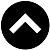-->

## PHYSICS FORM 1 SYLLABUS

1. Introduction To Physics
1. Concepts of Physics
1. Explain the concepts of physics
2. Establish the relationship between physics and other subjects
3. State the importance of studying physics
2. Applications of Physics in Real Life
1. Explain the applications of physics in real life
2. Apply physics in daily life
2. Introduction To Laboratory Practice
1. Laboratory Rules and Safety Guidelines
1. State rules in physics laboratory
2. Explain the safety measures in physics laboratory
3. Use the First Aid Kit to render first aid
4. Identify warning signs
5. Use warning signs in daily life
2. Basic Principles of Science Investigation
1. Explain the concept of scientific investigation
2. Identify the steps of scientific investigation
3. Use the scientific investigation methods in solving problems
3. Measurement
1. Concepts of Measurement
1. Explain the concepts of measurement
2. State the importance of measurement in real life
2. Basic Fundamental Quantities
1. Define a fundamental quantity
2. Mention three basic fundamental quantities of measurement
3. State the S.I unit of fundamental quantities
4. Use appropriate instruments for measuring fundamental quantities
3. Derived quantities
1. Explain derived quantities
2. State the S.I. units of derived quantities
4. Basic Apparatus/equipment's and their uses
1. Describe basic apparatus/equipments used for measurement
2. Identify sources of errors in measurement
5. Density and Relative Density
1. Explain the concept of density of a substance and its S.I unit
2. Determine the density of regular and irregular solids
3. Determine the density of a liquid
4. Define the relative density of a substance
5. Interpret applications of density and relative density in real life
4. Force
1. Concept of Force
1. Explain the concept of force
2. State the S.I unit of force
2. Types of Force
1. Identify fundamental types of forces
2. Describe the properties of each type of the fundamental forces
3. Effects of Force
1. Identify effects of forces
2. Justify the effects of forces on materials
5. Archimedes' Principle And Law Of Flotation
1. Archimedes' Principle
1. Explain the concept of upthrust
2. Verify the archimedes principle
3. Apply the archimedes principle to determine relative density
2. Law of Flotation
1. Distinguish floating and sinking of objects
2. Explain the conditions for a substance to float in fluids
3. Relate upthrust and weight of floating body
4. State the law of flotation
5. Apply the law of flotation in everyday life
6. Describe the mode of action of a Hydrometer
7. Construct a simple Hydrometer
8. Use Hydrometer to determine the relative density of different liquids
6. Structure And Properties Of Matter
1. Structure of Matter
1. Explain the concept of matter
2. Justify the particulate nature of matter
3. Explain the kinetic theory of matter
4. Classify three states of matter
2. Elasticity
1. Explain the concept of elasticity
2. Justify the relationship between tension and extension of a loaded elastic material
3. Identify the applications of elasticity in real life
1. Explain the concept adhesion and cohesion
2. Identify the applications of adhesion and cohesion in daily life
4. Surface Tension
1. Explain the concept of surface tension
2. Identify the applications of surface tension in daily life
5. Capillarity
1. Explain the concept of capillarity
2. Identify the applications of capillarity in daily life
6. Osmosis
1. Explain the concept of osmosis
2. Identify the applications of osmosis in daily life
7. Pressure
1. Concept of Pressure
1. Explain the concept of pressure
2. State the S.I unit of pressure
2. Pressure due to Solids
1. Explain dependence of pressure on surface of contact
2. Identify the applications of pressure due to solids
3. Pressure in Liquids
1. Describe the characteristics of pressure in liquids
2. Examine the variation of pressure with depth in liquids
3. Solve problems involving Pressure in Liquids
4. Explain the principle of a hydraulic pressure
5. Measure pressure of a liquid
4. Atmospheric Pressure
1. Describe the existence of atmospheric pressure
2. Identify the applications of atmospheric pressure
3. Measure atmospheric pressure
8. Work, Energy And Power
1. Work
1. Explain the concept of work
2. State the S.I unit of work
3. Determine the work done by an applied force
2. Energy
1. Explain the concept of energy
2. State S.I unit of energy
3. Identify different forms of energy
4. Distinguish between potential energy and kinetic energy
5. Explain the transformation of energy
6. State the principle of conservation of Energy
7. Explain the uses of mechanical energy
3. Power
1. Explain the concept of power
2. State the S.I unit of power
3. Determine the rate of doing work
9. Light
1. Sources of Light
1. Explain the concept of light
2. Identify sources of light
3. Distinguish luminous from non-luminous bodies
2. Propagation and Transmission of Light
1. Explain the concept of rays and beam of light
2. Verify that light rays travels in straight line
3. Identify transparent, translucent and opaque materials
3. Reflection of Light
1. Explain the concept of reflection of light
2. Distinguish regular from irregular reflection of light
3. Apply the laws of reflection of light
4. Describe image formed by a plane mirror